Next: B.2 Kernel PCA speed-up Up: B. Algorithms Previous: B. Algorithms

# B.1 Power method with deflation

The power method is a common method to extract the eigenvector with the largest eigenvalue (Diamantaras and Kung, 1996). Starting with a random vector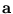, the principal eigenvector of a matrix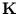is computed by iterating: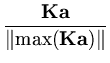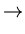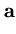. (B.1)

max() is the component of the vectorwith the largest absolute value (some variants of the power method use || instead). This iteration converges to the largest eigenvector with the eigenvalue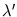= |max()|. Further eigenvectors are obtained using deflation. After the eigenvector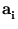(number i, ordered by the size of the corresponding eigenvalue) is computed, a new matrix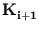is obtained from the previous one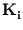by iterating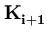=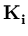-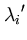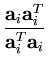, (B.2)

where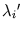is the eigenvalue corresponding to.Next: B.2 Kernel PCA speed-up Up: B. Algorithms Previous: B. Algorithms
Heiko Hoffmann
2005-03-22